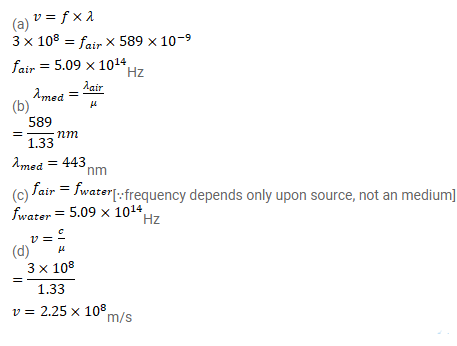# The wavelength of sodium light in air isQuestion:

The wavelength of sodium light in air is $589 \mathrm{~nm}$.

(a) Find its frequency in air.

(b) Find its wavelength in water (refractive index=1.33).

(c) Find its frequency in water.

(d) Find its speed in the water.

Solution: